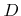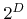Next: 5.2.2 Tangential Disks Up: 5.2 Ray Tracing Previous: 5.2 Ray Tracing

## 5.2.1 Surface Representation

The most common way to represent the surface is a line segmentation in case of two dimensions , or a triangulation in case of three dimensions [64,95]. The advantage of such explicit representations is that ray intersection tests are relatively simple for the surface elements (compare Appendix A). Furthermore, surface elements can be used as neighborhoods. For example, in  the particle flux is calculated for each triangle by counting the number of incident particles and dividing by the triangle area. However, if the surface is extracted using the marching cubes algorithm , the surface elements vary significantly in size. Hence, to obtain a good accuracy for all corresponding surface points, their neighborhoods must be extended over multiple surface elements, which requires an additional data structure and complicates the entire algorithm [A13].

Another drawback of the explicit surface representation is that it needs to be extracted from the implicit LS surface representation, which is needed for surface evolution using the LS method, at every time step. Hence, calculation time and memory are wasted due to this additional surface representation.

An improved strategy is to apply ray tracing directly on the implicit LS representation [112,A16]. To find the intersection of a ray with the surface, the LS function has to be bi- or trilinearly interpolated, depending on the number of dimensions. Inserting the parameterization of the ray into the interpolation formula gives a quadratic or cubic polynomial, respectively. Then the first real root, if it exists, corresponds to the intersection point. It is important for a fast ray tracing algorithm, that the intersection test requires a minimum number of numerical operations. In Appendix B a detailed and efficient ray-isosurface intersection test is presented.

Multi-linear interpolation within a grid cell requires the LS values of allgrid points. Hence, if the sparse field method is used, it must be ensured through dilation (see Section 4.3.3), that all these grid points are defined and their LS values are available.Next: 5.2.2 Tangential Disks Up: 5.2 Ray Tracing Previous: 5.2 Ray Tracing

Otmar Ertl: Numerical Methods for Topography Simulation